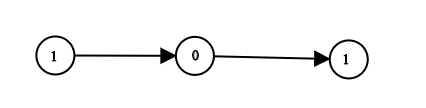### LeetCode - LinkedList - Convert Binary Number in a Linked List to Integer

Given `head` which is a reference node to a singly-linked list. The value of each node in the linked list is either 0 or 1. The linked list holds the binary representation of a number.

Return the decimal value of the number in the linked list.

Example 1:```Input: head = [1,0,1]
Output: 5
Explanation: (101) in base 2 = (5) in base 10```

```/**  * Definition for singly-linked list.  * public class ListNode {  *     int val;  *     ListNode next;  *     ListNode(int x) { val = x; }  * }  */ class Solution {     public int getDecimalValue(ListNode head) {         String num = "";         ListNode temp = head;         while(temp != null){             num = num+temp.val;             temp = temp.next;         }         //System.out.println(num);         int decimalValue = Integer.parseInt(num, 2);         return decimalValue;     } }```